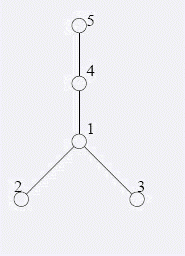# Bzoj1103 [poi2007]大都市meg

## 树的dfs序转化

Posted by yjjr's blog on November 17, 2018

# 题目

## Description

在经济全球化浪潮的影响下,习惯于漫步在清晨的乡间小路的邮递员Blue Mary也开始骑着摩托车传递邮件了。不过，她经常回忆起以前在乡间漫步的情景。昔日，乡下有依次编号为1..n的n个小村庄，某些村庄之间有一些双向的土路。从每个村庄都恰好有一条路径到达村庄1（即比特堡）。并且，对于每个村庄，它到比特堡的路径恰好只经过编号比它的编号小的村庄。另外，对于所有道路而言，它们都不在除村庄以外的其他地点相遇。在这个未开化的地方，从来没有过高架桥和地下铁道。随着时间的推移，越来越多的土路被改造成了公路。至今，Blue Mary还清晰地记得最后一条土路被改造为公路的情景。现在，这里已经没有土路了——所有的路都成为了公路，而昔日的村庄已经变成了一个大都市。 Blue Mary想起了在改造期间她送信的经历。她从比特堡出发，需要去某个村庄，并且在两次送信经历的间隔期间,有某些土路被改造成了公路.现在Blue Mary需要你的帮助：计算出每次送信她需要走过的土路数目。（对于公路，她可以骑摩托车；而对于土路，她就只好推车了。）

## Input

第一行是一个数n(1 < = n < = 2 50000).以下n-1行，每行两个整数a，b（1 < = a以下一行包含一个整数m（1 < = m < = 2 50000），表示Blue Mary曾经在改造期间送过m次信。以下n+m-1行，每行有两种格式的若干信息，表示按时间先后发生过的n+m-1次事件:若这行为 A a b(a若这行为 W a, 则表示Blue Mary曾经从比特堡送信到村庄a。

## Output

有m行，每行包含一个整数，表示对应的某次送信时经过的土路数目。

## Sample Input

5
1 2
1 3
1 4
4 5
4
W 5
A 1 4
W 5
A 4 5
W 5
W 2
A 1 2
A 1 3


## Sample Output

2
1
0
1


## HINTO(n)求出树的dfs序

# code

#include<iostream>
#include<cstdio>
#include<cstdlib>
#include<cmath>
#include<cstring>
#include<algorithm>
#define rep(i,a,b) for(int i=a;i<=b;i++)
#define dep(i,a,b) for(int i=a;i>=b;i--)
#define ll long long
#define mem(x,num) memset(x,num,sizeof x)
#define reg(x) for(int i=last[x];i;i=e[i].next)
using namespace std;
ll f=1,x=0;char ch=getchar();
while(ch<'0'||ch>'9'){if(ch=='-')f=-1;ch=getchar();}
while(ch>='0'&&ch<='9'){x=x*10+ch-'0';ch=getchar();}
return x*f;
}
const int maxn=250006;
int in[maxn],out[maxn],now,a[maxn<<1],last[maxn],n,m,cnt=0;
struct edge{int to,next;}e[maxn<<1];
void insert(int u,int v){e[++cnt]=(edge){v,last[u]};last[u]=cnt;}
void modify(int x,int val){for(int i=x;i<=n<<1;i+=i&(-i))a[i]+=val;}
inline int query(int x){int re=0;for(int i=x;i;i-=i&(-i))re+=a[i];return re;}
char ch;
void dfs(int x){
in[x]=++now;
modify(now,1);
reg(x){
if(in[e[i].to])continue;
dfs(e[i].to);
}
out[x]=++now;
modify(now,-1);
}
int main(){
rep(i,1,n-1){
insert(u,v);insert(v,u);
}
now=0;dfs(1);
rep(i,1,n+m-1){
scanf("%s",ch);
if(ch=='A'){